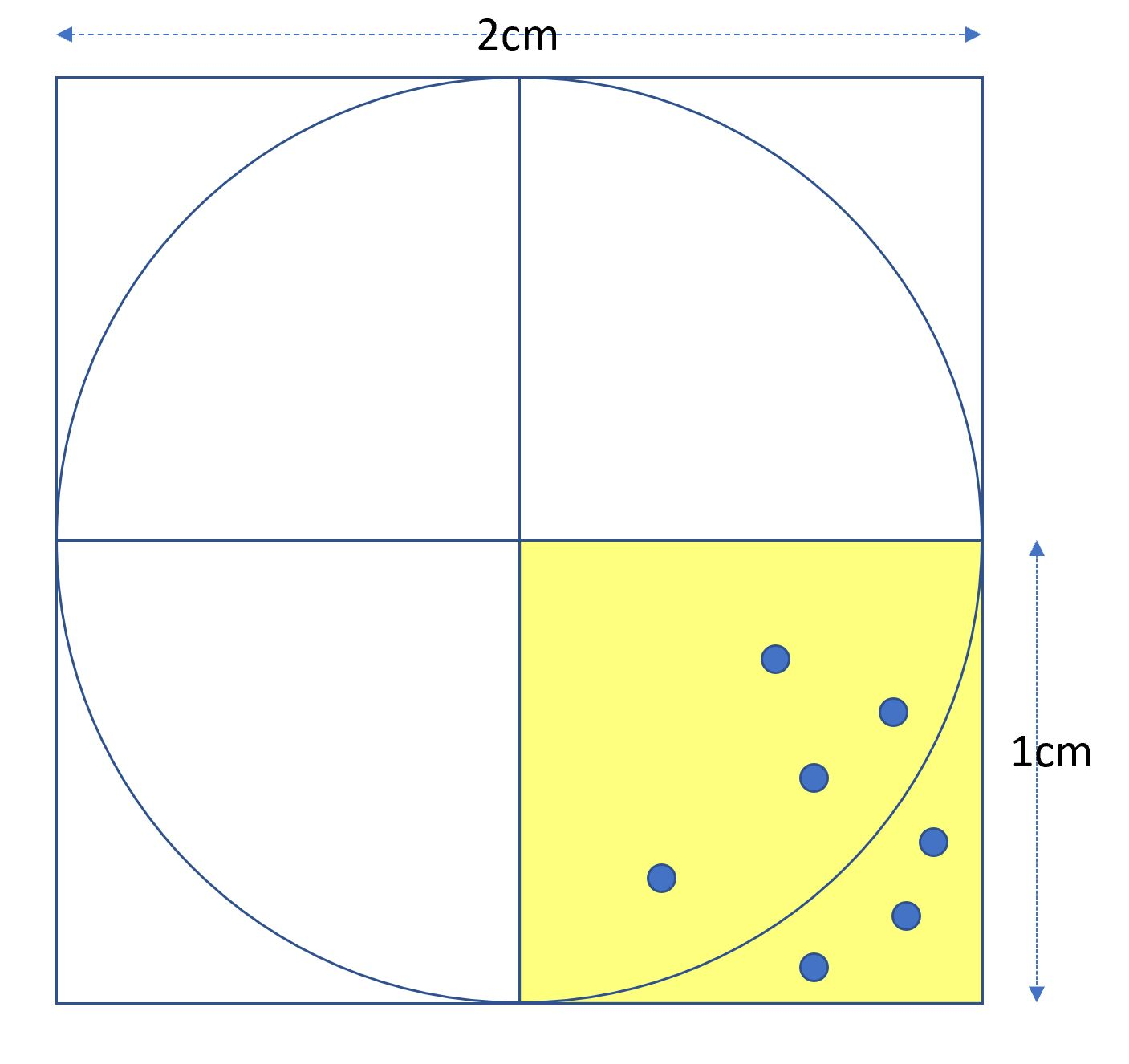# 蒙地卡羅方法

在〈蒙地卡羅方法〉中留言功能已關閉

# 原理import numpy as np
batch=100_000_000
epoch=200
incircle=0
for e in range(epoch):
t1=time.time()
points = [np.random.uniform(0, 1, batch), np.random.uniform(0, 1, batch)]
dist=np.sqrt(np.square(points)+np.square(points))
incircle+=np.where(dist<=1).shape
pi = incircle/((e + 1)*batch)*4
t2=time.time()
print(f"\rCPU Epoch:{e+1:03d} =>{t2-t1:.5f}秒, pi={pi}", end="")
結果:
CPU Epoch:004 =>1.95577秒, pi=3.14159577


CPU Epoch : 200 pi=3.1416053336


# GPU版本

GPU 一啟動，顯卡的溫度立即上升到攝氏 77 度，非常耗電。

import os
import time
os.environ['TF_CPP_MIN_LOG_LEVEL'] = '2'
import tensorflow as tf
#cpus = tf.config.list_physical_devices('CPU')
#tf.config.set_visible_devices (devices=cpus)
batch=100_000_000
b=tf.cast(batch, dtype=tf.float64)
epoch=500_000
incircle=0
pie=tf.constant(4, dtype=tf.float64)
@tf.function
def cal():
points=tf.random.uniform([2, batch], dtype=tf.float64)
dist=tf.sqrt(tf.square(points)+tf.square(points))
return dist

for e in range(epoch):
t1=time.time()
dist=cal()
count=tf.where(dist<=1).shape
incircle=incircle+ count
area = incircle * pie  / ((e + 1)*b)
t2=time.time()
print(f'\rGPU Epoch:{e+1:07,d} : {t2-t1:.5f}秒: pi={area}', end="")
結果:
GPU Epoch:0000167=>0.01596秒, pi=3.141591090778443
#CPU Epoch:0000005=>1.24264秒, pi=3.14158188


Epoch : 500,000 pi=3.1415926758672


# 改進

import os
import time

import numpy as np

os.environ['TF_CPP_MIN_LOG_LEVEL'] = '2'
import tensorflow as tf
batch=10_000_000
incircle=0
xs=tf.linspace(tf.cast(0., dtype=tf.float64),1., batch)
ys=tf.linspace(tf.cast(0., dtype=tf.float64),1., batch)
total_time1=time.time()
for i, x in enumerate(xs):
t1=time.time()
dist = tf.sqrt(tf.square(x) + tf.square(ys))
incircle=incircle+tf.where(dist<1).shape
pi=incircle/((i+1)*batch)*4
t2=time.time()
if i %100==0:
print(f"\rEpoch:{i:,}=>{t2-t1:.5f}秒, pi={pi}", end="")
print()
total_time2=time.time()
print(f"\r總花費:{total_time2-total_time1}秒, pi={pi}", end="")結果 :
Epoch:9,999,999=>0.00100秒, pi=3.1415924251788